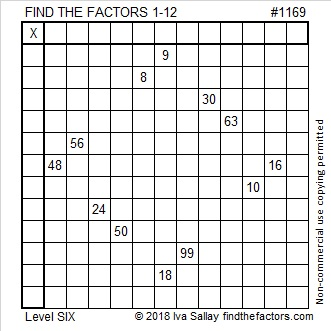# 1169 and Level 6

The twelve clues in this puzzle make an attractive puzzle for you to solve. What factors go with those clues? Can you find the logic needed to figure this one out?Print the puzzles or type the solution in this excel file: 12 factors 1161-1173

Now I’ll share what I’ve learned about the number 1169:

• 1169 is a composite number.
• Prime factorization: 1169 = 7 × 167
• The exponents in the prime factorization are 1 and 1. Adding one to each and multiplying we get (1 + 1)(1 + 1) = 2 × 2 = 4. Therefore 1169 has exactly 4 factors.
• Factors of 1169: 1, 7, 167, 1169
• Factor pairs: 1169 = 1 × 1169 or 7 × 167
• 1169 has no square factors that allow its square root to be simplified. √1169 ≈ 34.190641169 is the sum of consecutive prime numbers two different ways:
227+ 229 + 233+ 239+ 241 = 1169
383+ 389 + 397 = 1169

1169 is palindrome 5225 in BASE 6 because 5(6³) + 2(6²) + 2(6) + 5(1) = 1169

This site uses Akismet to reduce spam. Learn how your comment data is processed.